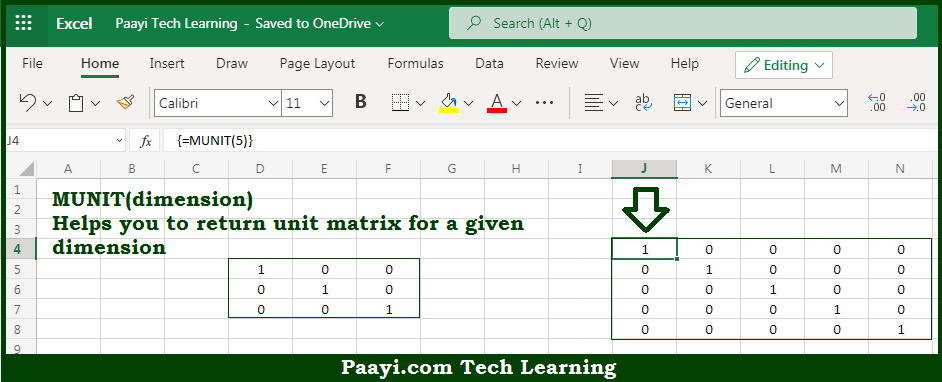# Learn How to Use Microsoft Excel MUNIT Function

Written by | 0 Comments | 544 Views

In this article, you will learn how to use the Microsoft Excel MUNIT function and its prime function in Microsoft Excel. You will also get to know the Microsoft Excel MUNIT function return value and syntax with the help of some examples.

Microsoft Excel MUNIT Function

The main function of the Microsoft Excel MUNIT function is to return the unit matrix for a given dimension. That implies, with the help of MUNIT function you can able to return the unit matrix for a given dimension, "n" which will be the size of n x n. It should be noted that the resulting unit matrix will have ones on the main diagonal and zeros in every other position. So, with the help of MUNIT function, you can able to get the unit matrix for a given dimension.

Return Value of MUNIT Function

The return value will be the unit matrix of size n x n.

Syntax of MUNIT Function

=MUNIT(dimension)

Where the arguments:

• dimension: This is the integer for the size of the unit matrix.

## How to Use Microsoft Excel MUNIT Function?So we know that Microsoft Excel MUNIT function you can able to return a unit matrix for a given dimension. That implies, with the help of MUNIT function you can able to return the unit matrix for a given dimension, "n" which will be the size of n x n. It should be noted that the resulting unit matrix will have ones on the main diagonal and zeros in every other position. So, with the help of MUNIT function, you can able to get the unit matrix for a given dimension.